# SAT Math Multiple Choice Question 237: Answer and Explanation

### Test Information

Question: 237

12. If csc θ = 1.66, then tan θ =

• A. 0.6
• B. 0.76
• C. 1.32
• D. 1.76

B Cosecant is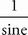or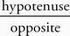. Make a right triangle and plug in for the side lengths. Because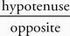, make the hypotenuse 1.66 and the side opposite the angle measure θ equal to 1. Use the Pythagorean theorem to solve for the missing side: 12 + x2 = 1.662; 1 + x2 = 2.7556; x2 = 1.7556; x ≈1.32. Make this the other leg of the triangle. To find tan θ, use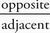: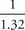, which is (B).